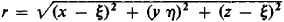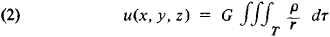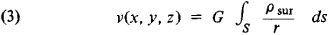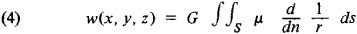# Potential

(redirected from brainstem auditory evoked potential)
Also found in: Dictionary, Thesaurus, Medical, Wikipedia.
Related to brainstem auditory evoked potential: auditory evoked responses, visual evoked potential, Somatosensory evoked potential

## potential

[pə′ten·chəl]
(electricity)
(physics)
A function or set of functions of position in space, from whose first derivatives a vector can be formed, such as that of a static field intensity.
McGraw-Hill Dictionary of Scientific & Technical Terms, 6E, Copyright © 2003 by The McGraw-Hill Companies, Inc.
The following article is from The Great Soviet Encyclopedia (1979). It might be outdated or ideologically biased.

## Potential

in the broad sense, the means, reserves, and sources that are available and capable of being mobilized, set in action, or used to attain a certain goal, carry out a plan, or solve some problem; the possibilities open to an individual, society, or state in a field, for example, economic potential or production potential.

## Potential

(also potential function), a concept characteristic of a broad class of physical force fields, such as electric and gravitational fields, and, in general, fields of any physical quantities representable by vectors, such as the velocity field in a fluid. For an electrostatic field, the potential is introduced as an auxiliary function whose spatial derivatives are the components of the electric field intensity at a given point. In hydrodynamics, they are the components of the velocity at a given point. In many cases, the potential also has another important physical meaning. Thus, in an electrostatic field it is numerically equal to the negative of the energy necessary to remove a unit positive charge from a given point to infinity.

In the general case of the potential of a vector field a(x, y, z), there is a scalar function u(x, y, z) such that a = grad u; that is, ax = ∂u/∂x, ay = ∂u/∂y, and az = ∂u/∂z, where ax, ay, and az are the components of field a in the Cartesian coordinate system Oxyz. If such a function can be introduced, then vector field a is called a potential field. In electrostatics, the potential can also be designated by the function U = — u. The potential of a vector field a is not determined unambiguously but only to the accuracy offactor. Therefore, only differences in potential between various points in the field are of interest in the study of a potential field. Geometrically, the equation u(x, y, z) = c represents a surface at all points of which the potential has the same value. Such surfaces are called equipotential surfaces.

For a gravitational field formed by a point mass m placed at point A(ξ, η, ξ), the potential, here called the Newtonian potential, at point P(x, y, z) has the form

(1) u(x, y, z) = Gm/r

whereand G is the gravitational constant. When fields are superposed on one another, their potentials are algebraically added. If a gravitational field is set up by some mass of density p(ξ, η, ξ) occupying a volume T, then it may be considered as a result of the superposition of the elementary fields formed by infinitesimally small bodies of mass ρdξdηdξ. The Newtonian potential of such a field is given by the integralThe potential u(x, y, z), together with its partial derivatives of the first order, is a continuous function throughout all space. Outside the body of volume T, the function u(x, y, z) satisfies the Laplace equation and, within the body, the Poisson equation.

If the attracting masses are distributed with a density psur over a surface S as a single layer, then the potential of the field formed by them is given by the integralThe potential of the single layer v(x, y, z) is a continuous function throughout all space outside of S. When the surface S is crossed, however, the normal derivative of the function v(x, y, z) experiences a discontinuity equal to 4πG/psur. By bringing together without limit two surfaces on which single layers with densities ρsur and —ρsur are located and by simultaneously increasing psur to infinity in such a way that the limit is lim npsur = μ, where η is the perpendicular distance between the surfaces, we reach the concept of the potential of a double layer:The potential of the double layer w(x, y, z) is a continuous function throughout all space outside of S. When the surface S is crossed, the function w(x, y, z) experiences a discontinuity, which is equal to 4πμ. The functions v(x, y, z) and w(x, y, z) both satisfy the Laplace equation.

If a body of volume T is an infinite cylinder of cross section D and if the density ρ of the matter in the cylinder is constant along every line parallel to the generatrices of the cylinder, then equation (2) leads to the concept of the logarithmic potential:

(5) u(x,y) = G ∫∫D ρ In (1/r) ds

Any harmonic function can be represented as the sum of the potentials of a single and double layer; this helps to explain the importance attached to potential theory.

### REFERENCES

Giunter, N. M. Teorii apotentsiala i eeprimenenie k osnovnym zadacham matematicheskoi fiziki. Moscow, 1953.
Sretenskii, L. N. Teorii n’iutonovskogo potentsiala. Moscow-Leningrad, 1946.
Tamm, I. E. Osnovy teorii elektrichestva, 7th ed. Moscow, 1957.
Idel’son, N. I. Teoriia potentsiala s prilozheniiami k teorii figury Zemli i geofizike, 2nd ed. Leningrad-Moscow, 1936.

V. I. BITIUTSKOV

References in periodicals archive ?
KEY WORDS: Brainstem Auditory Evoked Potentials; Absolute Latency; Interpeak Latency; Overt Hypothyroidism
Aging effects on brainstem auditory evoked potentials. Electromyogr Clin Neurophysiol.
Visual and brainstem auditory evoked potentials in children with obesity.
 However, no significant differences in the brainstem auditory evoked potentials in the progesterone phase compared with the estrogen phase was observed in study conducted on young normal females.
Scott, "Human brainstem auditory evoked potentials fail to provide evidence of efferent modulation of auditory input during attentional tasks," Psychophysiology, vol.
The diagnosis can be made by brainstem auditory evoked potentials and by gadolinium-enhanced magnetic resonance imaging (MRI).
Hearing screening of high-risk newborns with brainstem auditory evoked potentials: a follow-up study.
Brainstem auditory evoked potentials studies were performed in the electrodiagnostic unit under a standard protocol.
Furthermore Brainstem Auditory Evoked Potentials (BAEP) and Somatosensory Evoked Potentials (SSEP) remain abnormal throughout the course of the disease.
Jannetta, "Contribution from crossed and uncrossed brainstem structures to the brainstem auditory evoked potentials: a study in humans," Laryngoscope, vol.
 The electrophysiological testing reflects the bioelectric responses of the nervous system to sensory (somatosensory evoked potentials), auditory (brainstem auditory evoked potentials), or visual stimuli (visual evoked potentials).

Site: Follow: Share:
Open / Close